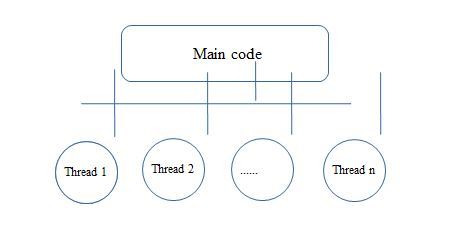Special Offer - Enroll Now and Get 2 Course at ₹25000/- Only Explore Now!# What is Multithreading in Python?

February 8th, 2020

• It simply means that the execution of two or more threads at the same time.
• The program can be split into multiple parts and these parts execute simultaneously, which in turn increases your program speed, performance and proper use of memory space.• Each of these threads is responsible for performing different tasks at the same time.
• Multithreading is useful for speeding up program execution and performance improvement, but it is not applied everywhere.
• It can be used only when there is no dependency between threads.
• Every program or process has one thread that will be running always, called the main thread.
• As the diagram shows child threads are created by the main thread.

### Example to create multiple threads

• Create a Thread class object in order to create a new thread that takes target and args as arguments.
• join() method can be used to wait until each thread is completely executed.
 ```# import the threading module import threading def add(num1,num2): """ function to print addition of given numbers """ result = num1+num2 print("Result is",result) def multiply(num1,num2): """ function to print multiplication of given numbers """ result = num1*num2 print("Result is",result) if __name__ == "__main__": # creating th1 = threading.Thread(target=add, args=(10,20)) th2 = threading.Thread(target=multiply, args=(10,20)) # th 1 starts th1.start() # th 2 starts th2.start() # wait until th 1 is executed completely th1.join() # wait until th 2 is executed completely th2.join() # both threads completely executed print("Thread Completed!")```

#### Output of above program is:

 ```Result is 30 Result is 200 Done!```

### List of functions available in thread module

start()
To start each thread. only one start() function is used for each thread.
run()
This defines activity of the thread To override the function of the class that extends the thread class.
join()
It waits until completion of each thread and then starts other thread execution.
activeCount()
It gives count of active threads
It returns object of current thread class.
enumerate()
It returns list of active thread objects.
isAlive()
It returns true, if the thread is alive

### Program to get current thread name

 ```import threadingdef thread1(): print("thread 1 assigned to thread: {}".format(threading.current_thread().name)) def thread2(): print("thread 2 assigned to thread: {}".format(threading.current_thread().name)) if __name__ == "__main__": # creating th1 = threading.Thread(target=thread1, name='th1') th2 = threading.Thread(target=thread2, name='th2') # starting threads th1 and th2 th1.start() th2.start() # wait until all threads finish th1.join() th2.join()```

#### Output:

 ```thread 1 assigned to thread: th1 thread 2 assigned to thread: th2```